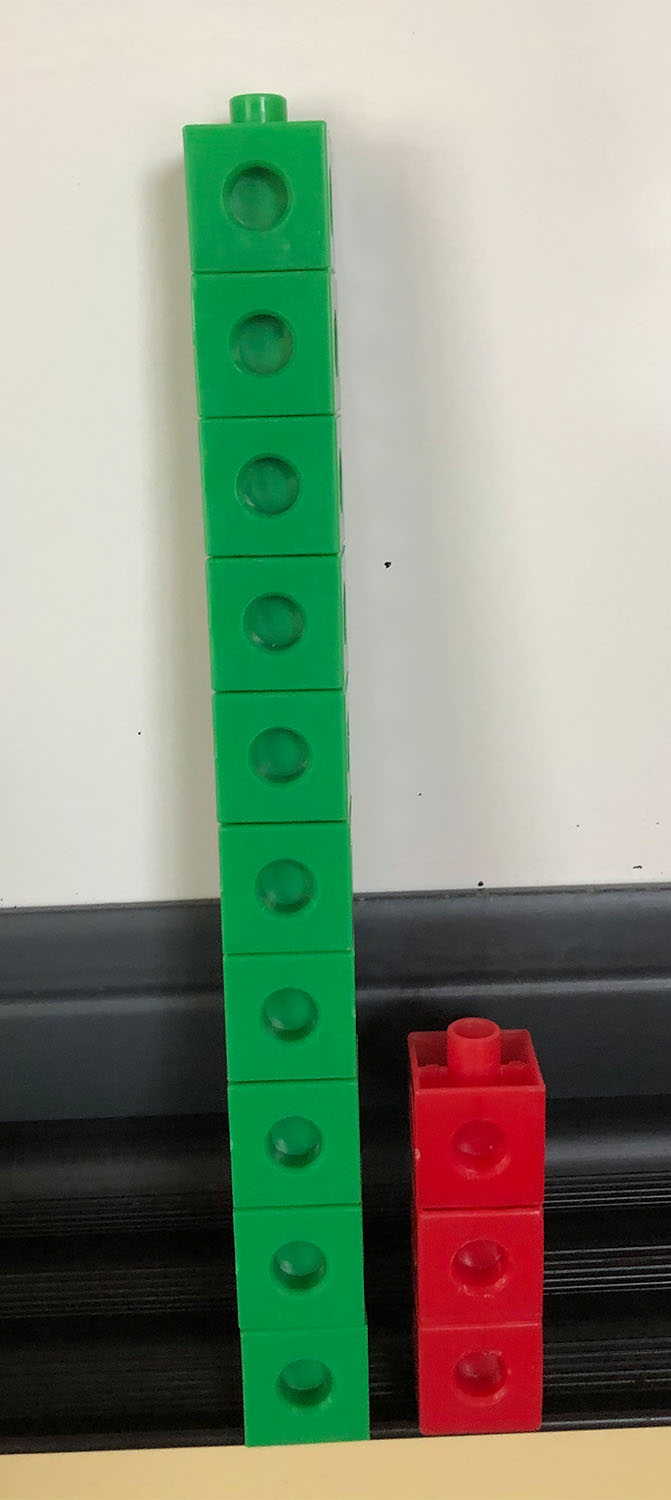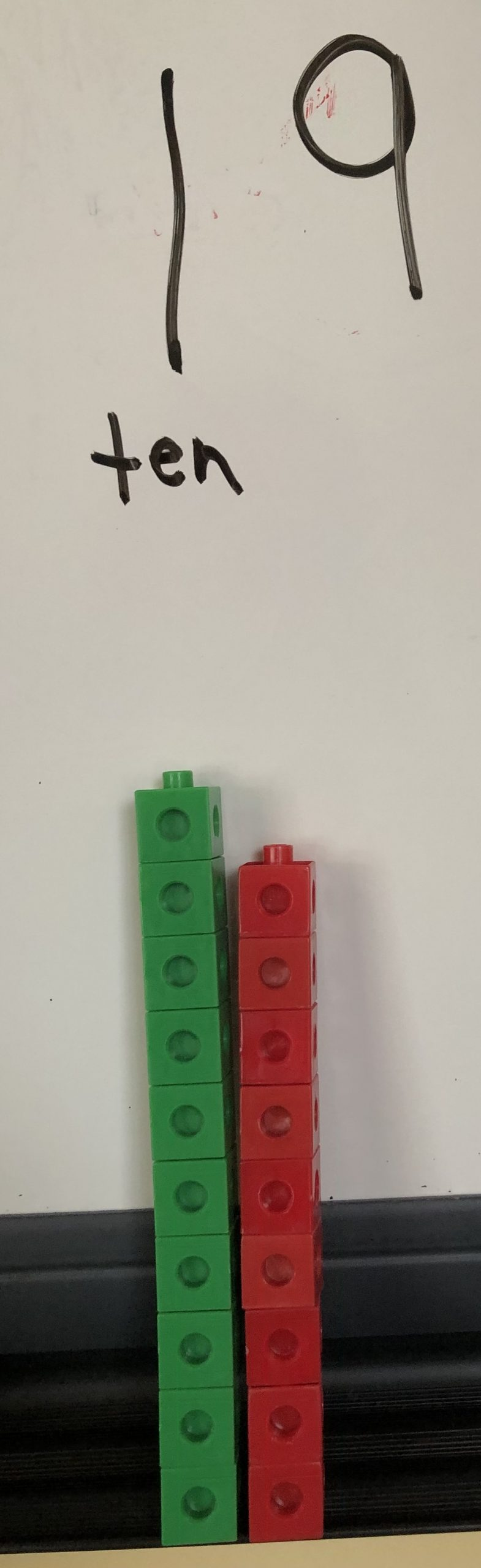Select Page

What follows is a lightly edited version of the second part of an end-of-Kindergarten discussion about the teen numbers. In Part 1, students took up the question of whether 100 is a teen number. That conversation built on a previous one, about why there are so many 1s in the teen numbers.

At a certain point in the conversation about whether 100 is a teen number, the discussion began circling around the same ideas, ones that had already been shared. At that point, the teacher made a decision to highlight one student’s idea, and ask the class to think about it.

Let’s go back to something I heard Isaiah say. Isaiah said [pointing to the number line] “10 is 10 plus zero and that makes 10.” [She writes 10+0=10 on the board.]

Pablo: He said 10 + 0 equals a teen number.

You’re right. He said 10+0 equals a teen number. Why does 10+0 equal a teen number?

Stella: Because 10 is a teen number.

Are there any other numbers that if we add 10 to it, it would make a teen number?

Pablo: 10 + 9 would be 19. [The teacher records 10+9=19.] 100 looks like it’s a teen number but it isn’t basically actually because it isn’t with the group.

I hear people saying if you’re going to be a teen number you have to be with the group. [Other students also made this argument in Part 1 of the discussion.]

Isaiah: But it does start with a one so it is technically a teen number?

Pablo: Because it’s one hundred so it has to start with a one.

Cara: The one is supposed to be there because it’s one hundred.

And if it were two hundred, what would that number start with?

Pablo: A two.

So, what does the one at the start of these teen numbers mean? [Long wait time.] Listen to that question again. What does the one at the start of these teen numbers mean? Someone said the one at the start of 100 means one hundred. So then what does the one at the start of a teen number mean?

Nicole: I think it represents that it’s a teen number so then people know that that’s a teen number.

Pablo agrees. The teacher takes out some cubes:What number do I have there?

Various: “3?” “No, not a 3.” “It’s a 10 and a 3.” “It’s 13.” “It’s a 13!

Alli: It’s a 10 and a 3.

Pablo: It’s a 13 because if you have a 10 and then a 3, 10 plus 3 equals 13.

Do we agree that that’s 13, because when we add 10 plus 3 together it’s 13? [She writes 13 on the board.] So, thinking about these two towers that I have here, what does the 1 in 13 represent?

Nicole: It represents the 10 and then 3 more.

Did you hear what Nicole said? She said the 1 represents the 10 and the 3 represents what? The 3 more. … Will that work for all the teen numbers?

Pablo: No. … Wait! Yes it will! It doesn’t all end with a 3 but if you add in more red ones, for like if it’s 19, there will be 9 in that tower.

The teacher builds 19.

So how many do I have here? (19!) What does the 1 represent in 19?

Isaiah: The 1 represents the 10 and the 9 represents the 9 and, but 10+9 also equals 19.

I want to look at that one more time. I have a tower of 10 and I have a tower of 9. And we decided that this is how many? (19) [She writes 19 on the board.] So what does the 1 represent

Pablo: The 10! but then the 9 represents the 9.

So I have one tower of 10 [she adds the word “ten” beneath the 19]…Pablo: And then the 9 represents the 9 because that’s how you do it, 10+9=19.

Isaiah: Like I said, those 2 numbers represent different numbers but [he makes a combining gesture with his hands] the same number as what those two numbers would equal.

So the two numbers represent the amount in each of my towers.

Isaiah: And those two amounts would equal that number!

This teacher has created an environment where mathematical thinking thrives. She also did a lot of thoughtful, careful work in-the-moment; work that may be hard to see. Given the difficulty of the place value ideas embedded in understanding the way we write 100, her goal of getting to the big kindergarten idea (i.e. the teen numbers are comprised of 10 ones and some number of ones), and the repetition of the ideas being shared, she made an important decision in the middle of this discussion (i.e. at the end of Part 1/the beginning of this blog).

She decided to try to move the discussion forward and in a slightly different direction. She posed questions that asked kids to consider and extend particular ideas, ideas that had been voiced by their classmates, ideas that focused them on the important mathematics in the teen numbers. And, she introduced a representation that did the same.

Her ability to do this so effectively depends on her knowledge of:

• the math at hand (i.e. the 1 in 100 stands for 100 ones or 1 hundred)
• the related Kindergarten math (i.e. the 1 in the teen numbers stands for 10 ones or 1 ten)
• the challenges inherent of making sense of those ideas (i.e. thinking of 10 ones as 1 ten requires using two different numbers to describe the same quantity, to count objects and groups, simultaneously; in the base-10 system, the place of a digit dictates its value; and, the way we name the teen numbers—eleven, twelve instead of ten-one, ten-two—obscures the idea that there is one ten in each number)
• representations that are familiar to her students (e.g. cubes) that might best illustrate and highlight the important math ideas.

As I reflect on this conversation, I keep thinking about the decisions this teacher made and the impact they had. She pursued students’ question about 100 as long as it seemed productive, then asked them to consider Isaiah’s idea that “10+0 equals a teen number, 10.” After discussing the 1 in 100 and the 2 in 200, she poses her own question: “What does the one at the start of these teen numbers mean?” These questions helped focus the conversation on the composition of the teen numbers, and the discussion really took off when cube representations of the teen numbers were introduced. For these students, most of whom are still counting by 1s, the cube representation is what helps them see something important about the way we write the teen numbers. They are connecting the cubes (i.e. a tower of 10 and a tower of 9) to the symbols (i.e. 19), and are thinking about those ten cubes as important, and as connected to the 1 in each of the teen numbers. Quite an achievement. One they will continue to build on in the years to come.

Tag(s): Kindergarten | place value | teen numbers |